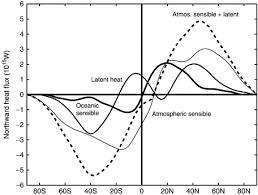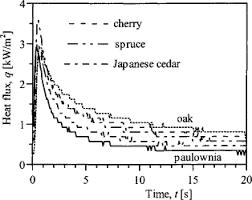## How to Calculate and Solve for Heat Flux | Solidification of MetalsThe image above represents heat flux.

To compute for heat flux, three essential parameters are needed and these parameters are Heat Emission (h), Surface Temperature (Ts) and Initial Temperature (To).

The formula for calculating heat flux:

qx = h(Ts – To)

Where:

qx = Heat Flux (T)
h = Heat Emission
Ts = Surface Temperature
To = Initial Temperature

Let’s solve an example;
Find the heat flux when the heat emission is 21, the surface temperature is 12 and the initial temperature is 10.

This implies that;

h = Heat Emission = 21
Ts = Surface Temperature = 12
To = Initial Temperature = 10

qx = h(Ts – To)
qx = 21(12 – 10)
qx = 21(2)
qx = 42

Therefore, the heat flux is 42 W.

Calculating the Heat Emission when the Heat Flux, the Surface Temperature and the Initial Temperature is Given.

h = qx / Ts – To

Where:

h = Heat Emission
qx = Heat Flux (T)
Ts = Surface Temperature
To = Initial Temperature

Let’s solve an example;
Find the heat emission when the heat flux is 40, the surface temperature is 20 and the initial temperature is 10.

This implies that;

qx = Heat Flux (T) = 40
Ts = Surface Temperature = 20
To = Initial Temperature = 10

h = qx / Ts – To
h = 40 / 20 – 10
h = 40 / 10
h = 4

Therefore, the heat emission is 4.

## How to Calculate and Solve for Heat Flux | Solidification of MetalsThe image above represents heat flux.

To compute for heat flux, three essential parameters are needed and these parameters are Thermal Conductivity (K), Density (ρ) and Heat Capacity at Constant Pressure (cp).

The formula for calculating heat flux:

q = K / ρcp

Where:

q = Heat Flux
K = Thermal Conductivity
ρ = Density
cp = Heat Capacity at Constant Pressure

Let’s solve an example;
Find the heat flux when the thermal conductivity is 21, the density is 12 and the heat capacity at constant pressure is 24.

This implies that;’

K = Thermal Conductivity = 21
ρ = Density = 12
cp = Heat Capacity at Constant Pressure = 24

q = K / ρcp
q = 21 / (12)(24)
q = 21 / 288
q = 0.0729

Therefore, the heat flux is 0.0729 W.

Calculating the Thermal Conductivity when the Heat Flux, the Density and the Heat Capacity at Constant Pressure is Given.

K = q (ρ cp)

Where:

K = Thermal Conductivity
q = Heat Flux
ρ = Density
cp = Heat Capacity at Constant Pressure

Let’s solve an example;
Find the thermal conductivity when the heat flux is 15, the density is 10 and the heat capacity at constant pressure is 14.

This implies that;

q = Heat Flux = 15
ρ = Density = 10
cp = Heat Capacity at Constant Pressure = 14

K = q (ρ cp)
K = 15 (10 x 14)
K = 15 (140)
K = 2100

Therefore, the thermal conductivity is 2100.